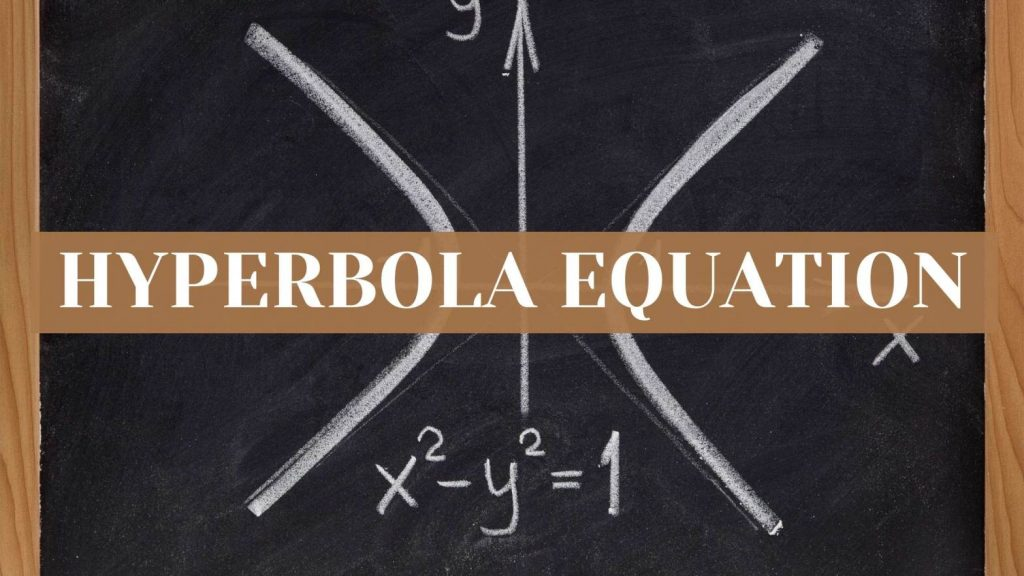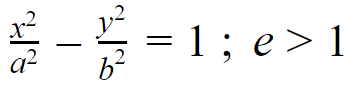Maths
Study Material

# Hyperbola Equation

A hyperbola represents the locus of the points, the difference of whose distances from the two foci (the two fixed points) of the hyperbola is a constant value.

6 minutes long
Posted by Kunduz Tutor, 14/11/2021Hesap Oluştur

Got stuck on homework? Get your step-by-step solutions from real tutors in minutes! 24/7. Unlimited.

Hyperbola is an important topic of the conic section which comprises a major portion of the conic section. Hyperbola Equation represents a smooth curve in a plane, and it shares a number of similarities with an ellipse (concepts like foci, center, eccentricity, directrix, latus rectum, etc.).

We can understand hyperbola by considering some simple real-life examples of the hyperbola in our daily lives; the path that is followed by the tip of the shadow of a sundial, the shadow of a lampshade or a flashlight, the scattering trajectory of sub-atomic particles, etc.

Author – Ojasvi Chaplot

## Hyperbola Meaning

A hyperbola represents the locus of the points, the difference of whose distances from the two foci (the two fixed points) of the hyperbola is a constant value.

In other words, a hyperbola can be described as the locus of a point moving in a plane such that the ratio of its distance from the focus(a fixed point) to that from the directrix(a fixed line) is a constant value which is greater than 1.

## Basic Terms of a Hyperbola

The general terms (or terminologies) in relation to the hyperbola and hyperbola equation(s) are explained below:

### Foci of Hyperbola

• The two fixed points of the hyperbola are called as the foci (plural of focus) of the hyperbola. The distance between the foci is equal to 2c; where, c = ae; here, e is the eccentricity.

### Eccentricity of Hyperbola

• The eccentricity of a hyperbola is greater than one (i.e. e > 1). Eccentricity of a hyperbola is the ratio of the distances from the center of the hyperbola to one of the foci and to one of the vertices of the hyperbola. The eccentricity is also known as the degree of flatness.

### Center of Hyperbola

• The mid-point of the line segment joining the foci is called the center of the hyperbola.

### Vertices of a Hyperbola

• The points at which the hyperbola intersects the transverse axis are called the vertices of the ellipse. The distance between the two vertices is equal to 2a.

### Transverse Axis of Hyperbola

• The line segment through the foci of the hyperbola is called as the transverse axis. The length of the transverse axis is given by 2a.

### Conjugate Axis of Hyperbola

•  The line segment through the center and perpendicular to the transverse axis is called the conjugate axis. The length of the conjugate axis is given by 2b.

### Latus Rectum of Hyperbola

• The latus rectum of the hyperbola is a line segment perpendicular to the transverse axis through any of the foci and whose end points lie on the hyperbola.

## Hyperbola Equation

The general equation of the hyperbola can be used to represent a hyperbola algebraically in the coordinate plane. The hyperbola equation can be written as,## Hyperbola Formulas

The general equation of hyperbola and the various properties and formulas related to the hyperbola equation are explained through the table given below–

## Hyperbola Graph

For representing on a graph, we can say that hyperbola is a mix of two parabolas — each one is a perfect mirror image of the other, and each opening away from one another. Hyperbolic curve are generally of two types:

• Horizontal and
• Vertical.

## Derivation of Hyperbola Equation

The derivation of the hyperbola equation can be shown as follows:

Let’s consider any point P (x, y) on the hyperbola and according to the definition of the hyperbola, the difference of its distances from each of the two foci F and F’ (the two fixed points) of the hyperbola is equal to 2a.

• PF – PF’ = 2a

Now, by using the distance formula,

we have,

• √{(x + c)2 + y2} – √{(x – c)2 + y2} = 2a
• √{(x + c)2 + y2} = 2a + √{(x – c)2 + y2}

Further, on squaring both the sides, we get

• (x + c)2 + y2 = 4a2 + 4a√{(x – c)2 + y2} + (x – c)2 + y2

On simplifying the above equation, we get

• 4cx – 4a2 = 4a√{(x – c)2 + y2}
• cx – a2 = √{(x – c)2 + y2}

Again, on squaring both the sides and simplifying it further, we get

• x2/a2 + y2/(a2 – c2) = 1
• x2/a2 – y2/(c2 – a2) = 1

As we know that, c2 = a2 + b2

Therefore, on substituting it, we get the result as

• x2/a2 – y2/b2 = 1

Thus, this derives the standard hyperbola equation: x2/a2 – y2/b2 = 1

## Special Cases of Hyperbola Equation

• If e1 and e2 are the eccentricities standard and its conjugate hyperbola, then (1/e12) + (1/e22) = 1
• If the two hyperbolas have the same eccentricities, then they are said to be similar.
• The two similar hyperbolas are said to be equal if they have the same latus rectum.
• A hyperbola in which if a = b, then it is called as an equilateral hyperbola.
• A hyperbola in which if a = – b, then it is the case of a rectangular hyperbola and its eccentricity is equal to √2.

## Picked For You

Furthermore, these are some topics which might interest you: